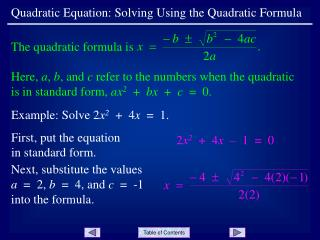DownloadDownload PresentationQuadratic Equation: Solving Using the Quadratic Formula

# Quadratic Equation: Solving Using the Quadratic Formula

Télécharger la présentation## Quadratic Equation: Solving Using the Quadratic Formula

- - - - - - - - - - - - - - - - - - - - - - - - - - - E N D - - - - - - - - - - - - - - - - - - - - - - - - - - -
##### Presentation Transcript

1. The quadratic formula is Quadratic Equation: Solving Using the Quadratic Formula Here, a, b, and c refer to the numbers when the quadratic is in standard form, ax2 + bx + c = 0. Example: Solve 2x2 + 4x = 1. First, put the equation in standard form. 2x2 + 4x – 1 = 0 Next, substitute the values a = 2, b = 4, and c = -1 into the formula.

2. These are the exact solutions. Approximate solutions can be found by separately evaluating and to get approximately - 2.225 and 0.225. Quadratic Equation: Solving Using the Quadratic Formula Next, simplify. Slide 2

3. Quadratic Equation: Solving Using the Quadratic Formula Try: Solve x2 + 5 = - 2x. The solutions are - 1  2i. The quadratic formula may be programmed into your calculator. See your instructor to obtain the program and instructions for running it. Slide 3

4. Quadratic Equation: Solving Using the Quadratic Formula END OF PRESENTATION Click to rerun the slideshow.